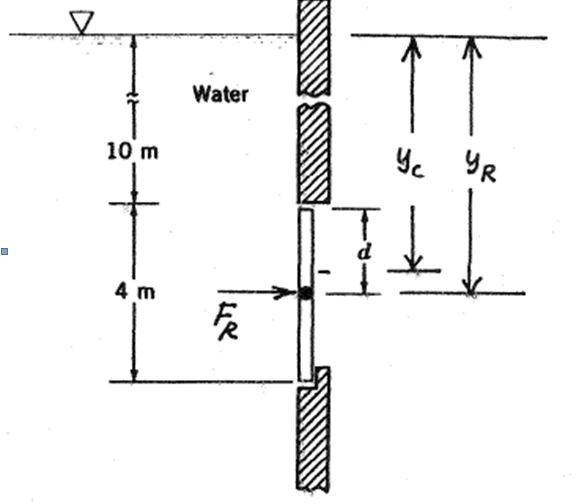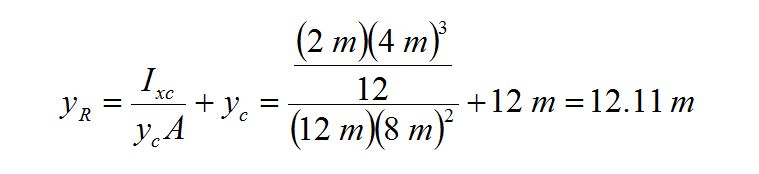## Vibrations and ControlsIn this problem the horizontal shaft is located in the middle of the gate. Part of the gate is above the shaft and part is below it. If the net pressure below the location of the shaft is greater than that above the shaft the gate will remain closed because of the block on the right side of the bottom of the gate. However, if the net pressure above the shaft is greater than the net pressure below the shaft, the gate will open because there is no blockage in the gate.the figure at the top shows the gate with the coordinates of the centroid and the the center of pressure. (In this figure yCP is denoted as yR.) If the hinge is located at the center of pressure, yR, the net pressure forces on the top and bottom of the shaft will be exactly the same. Any increase in depth will cause the gate to open.

In the figure as shown the coordinate of the centroid of the gate, yc = 10 m + 2 m = 12 m relative to the surface of the liquid. The area of the gate is (4 m)(2 m) = 8 m2. The 4 m side is in the vertical direction that is used in the formula for the moment of inertia about the centroid: Ixc = (horizontal side) (vertical side) / 12. Using the equation for the center of pressure gives the following resultFrom the diagram we see that the location of yR equals d + 10 m so that d = yR

Go To Top 回顶部

QQ客服